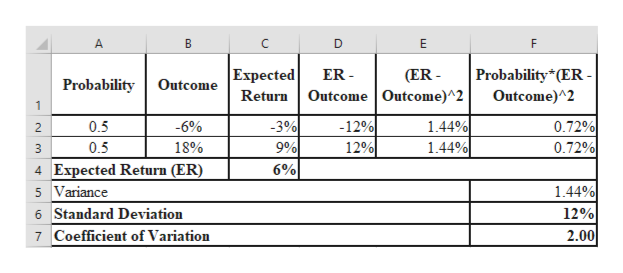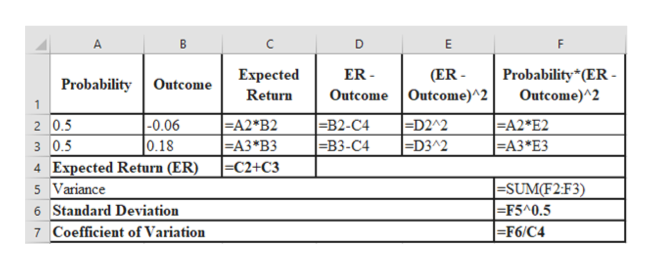# You are given the following probability distribution for a stock:Probability                   Outcome .5                                -6%.5                                 18%A) Compute the expected return.B) Compute the standard deviation.C) Compute the coefficient of variation.

Question
17 views

You are given the following probability distribution for a stock:

Probability                   Outcome

.5                                -6%
.5                                 18%

A) Compute the expected return.
B) Compute the standard deviation.
C) Compute the coefficient of variation.

check_circle

Step 1

Calculation of expected return, standard deviation and coefficient of variation using excel is as follows:help_outlineImage TranscriptioncloseВ D E F Probability (ER- ER Outcome Outcome)^2 Expected (ER- Probability Outcome Return Outcome)^2 1 0.72% 0.72% -3% 9% 6% -12% 12% 144% 1.44% 0.5 -6% 2 18% 0.5 4 Expected Return (ER) 5 Variance 1.44% 12% 2.00 6 Standard Deviation Coefficient of Variation 7 fullscreen
Step 2

Excel formula used in the abov...help_outlineImage TranscriptioncloseВ F Probability (ER- Outcome)^2 (ER Outcome Outcome)^2 Expected ER Probability Outcome Return 1 2 0.5 з 0.5 4 Expected Return (ER) =D2^2 =D3^2 -0.06 0.18 A2*B2 |-АЗ*ВЗ C2+C3 -B2-С4 |ЕВ3-С4 A2 E2 |ЕА3 "ЕЗ |=SUM(F2:F3) =F5^0.5 =F6/C4 5 Variance 6 Standard Deviation Coefficient of Variation 7 fullscreen

### Want to see the full answer?

See Solution

#### Want to see this answer and more?

Solutions are written by subject experts who are available 24/7. Questions are typically answered within 1 hour.*

See Solution
*Response times may vary by subject and question.
Tagged in